# Discrete compartmental models in a nutshell

## From continuous to discrete time

In its simplest form, a SIR model is typically written in continuous time as:

$\frac{dS}{dt} = - \beta \frac{S_t I_t}{N_t}$

$\frac{dI}{dt} = \beta \frac{S_t I_t}{N_t} - \gamma I_t$

$\frac{dR}{dt} = \gamma I_t$

Where $$\beta$$ is an infection rate and $$\gamma$$ a removal rate, assuming ‘R’ stands for ‘recovered’, which can mean recovery or death.

For discrete time equivalent, we take a small time step $$t$$ (typically a day), and write the changes of individuals in each compartment as:

$S_{t+1} = S_t - \beta \frac{S_t I_t}{N_t}$

$I_{t+1} = I_t + \beta \frac{S_t I_t}{N_t} - \gamma I_t$

$R_{t+1} = R_t + \gamma I_t$

## Stochastic processes

The discrete model above remains deterministic: for given values of the rates $$\beta$$ and $$\gamma$$, dynamics will be fixed. It is fairly straightforward to convert this discrete model into a stochastic one: one merely needs to uses appropriate probability distributions to model the transfer of individuals across compartments. There are at least 3 types of such distributions which will be useful to consider.

### Binomial distribution

This distribution will be used to determine numbers of individuals leaving a given compartment. While we may be tempted to use a Poisson distribution with the rates specified in the equations above, this could lead to over-shooting, i.e. more individuals leaving a compartment than there actually are. To avoid infecting more people than there are susceptibles, we use a binomial distribution, with one draw for each individual in the compartment of interest. The workflow will be:

1. determine a per-capita probability of leaving the compartment, based on the original rates specified in the equations; if the rate at which each individual leaves a compartment is $$\lambda$$, then the corresponding probability of this individual leaving the compartment in one time unit is $$p = 1 - e^{- \lambda}$$.

2. determine the number of individuals leaving the compartment using a Binomial distribution, with one draw per individual and a probability $$p$$

As an example, let us consider transition $$S \rightarrow I$$ in the SIR model. The overall rate at which this change happens is $$\beta \frac{S_t I_t}{N_t}$$. The corresponding per susceptible rate is $$\beta \frac{I_t}{N_t}$$. Therefore, the probability for an individual to move from S to I at time $$t$$ is $$p_{(S \rightarrow I), t} = 1 - e^{- \beta \frac{I_t}{N_t}}$$.

### Poisson distribution

Poisson distributions will be useful when individuals enter a compartment at a given rate, from ‘the outside’. This could be birth or migration (for $$S$$), or introduction of infections from an external reservoir (for $$I$$), etc. The essential distinction with the previous process is that individuals are not leaving an existing compartment.

This case is simple to handle: one just needs to draw new individuals entering the compartment from a Poisson distribution with the rate directly coming from the equations.

For instance, let us now consider a variant of the SIR model where new infectious cases are imported at a constant rate $$\epsilon$$. The only change to the equation is for the infected compartment:

$I_{t+1} = I_t + \beta \frac{S_t I_t}{N_t} + \epsilon - \gamma I_t$

where:

• individuals move from $$S$$ to $$I$$ according to a Binomial distribution $$\mathcal{B}(S_t, 1 - e^{- \beta \frac{I_t}{N_t}})$$

• new infected individuals are imported according to a Poisson distribution $$\mathcal{P}(\epsilon)$$

• individual move from $$I$$ to $$R$$ according to a Binomial distribution $$\mathcal{B}(I_t, 1 - e^{- \gamma})$$

### Multinomial distribution

This distribution will be useful when individuals leaving a compartment are distributed over several compartments. The Multinomial distribution will be used to determine how many individuals end up in each compartment. Let us assume that individuals move from a compartment $$X$$ to compartments $$A$$, $$B$$, and $$C$$, at rates $$\lambda_A$$, $$\lambda_B$$ and $$\lambda_C$$. The workflow to handle these transitions will be:

1. determine the total number of individuals leaving $$X$$; this is done by summing the rates ($$\lambda = \lambda_A + \lambda_B + \lambda_C$$) to compute the per capita probability of leaving $$X$$ $$(p_(X \rightarrow ...) = 1 - e^{- \lambda})$$, and drawing the number of individuals leaving $$X$$ ($$n_{_(X \rightarrow ...)}$$) from a binomial distribution $$n_{(X \rightarrow ...)} \sim B(X, p_(X \rightarrow ...))$$

2. compute relative probabilities of moving to the different compartments (using $$i$$ as a placeholder for $$A$$, $$B$$, $$C$$): $$p_i = \frac{\lambda_i}{\sum_i \lambda_i}$$

3. determine the numbers of individuals moving to $$A$$, $$B$$ and $$C$$ using a Multinomial distribution: $$n_{(X \rightarrow A, B, C)} \sim \mathcal{M}(n_{(X \rightarrow ...)}, p_A, p_B, p_C)$$

# Implementation using odin

## Deterministic SIR model

We start by loading the odin code for a discrete, stochastic SIR model:

path_sir_model <- system.file("examples/discrete_deterministic_sir.R", package = "odin")
## Core equations for transitions between compartments:
update(S) <- S - beta * S * I / N
update(I) <- I + beta * S * I / N - gamma * I
update(R) <- R + gamma * I

## Total population size (odin will recompute this at each timestep:
## automatically)
N <- S + I + R

## Initial states:
initial(S) <- S_ini # will be user-defined
initial(I) <- I_ini # will be user-defined
initial(R) <- 0

## User defined parameters - default in parentheses:
S_ini <- user(1000)
I_ini <- user(1)
beta <- user(0.2)
gamma <- user(0.1)

As said in the previous vignette, remember this looks and parses like R code, but is not actually R code. Copy-pasting this in a R session will trigger errors.

We then use odin to compile this model:

sir_generator <- odin::odin(path_sir_model)
## Loading required namespace: pkgbuild
sir_generator
## <odin_model> object generator
##   Public:
##     initialize: function (..., user = list(...), use_dde = FALSE, unused_user_action = NULL)
##     ir: function ()
##     set_user: function (..., user = list(...), unused_user_action = NULL)
##     initial: function (step)
##     rhs: function (step, y)
##     update: function (step, y)
##     contents: function ()
##     transform_variables: function (y)
##     run: function (step, y = NULL, ..., use_names = TRUE)
##   Private:
##     ptr: NULL
##     use_dde: NULL
##     odin: NULL
##     variable_order: NULL
##     output_order: NULL
##     n_out: NULL
##     ynames: NULL
##     interpolate_t: NULL
##     cfuns: list
##     dll: discrete.deterministic.sir7184bd4d
##     user: I_ini S_ini beta gamma
##     registration: function ()
##     set_initial: function (step, y, use_dde)
##   Parent env: <environment: namespace:discrete.deterministic.sir7184bd4d>
##   Locked objects: TRUE
##   Locked class: FALSE
##   Portable: TRUE

Note: this is the slow part (generation and then compilation of C code)! Which means for computer-intensive work, the number of times this is done should be minimized.

The returned object sir_generatoris an R6 generator that can be used to create an instance of the model: generate an instance of the model:

x <- sir_generator$new() x ## <odin_model> ## Public: ## contents: function () ## initial: function (step) ## initialize: function (..., user = list(...), use_dde = FALSE, unused_user_action = NULL) ## ir: function () ## rhs: function (step, y) ## run: function (step, y = NULL, ..., use_names = TRUE) ## set_user: function (..., user = list(...), unused_user_action = NULL) ## transform_variables: function (y) ## update: function (step, y) ## Private: ## cfuns: list ## dll: discrete.deterministic.sir7184bd4d ## interpolate_t: NULL ## n_out: 0 ## odin: environment ## output_order: NULL ## ptr: externalptr ## registration: function () ## set_initial: function (step, y, use_dde) ## update_metadata: function () ## use_dde: FALSE ## user: I_ini S_ini beta gamma ## variable_order: list ## ynames: step S I R x is an ode_model object which can be used to generate dynamics of a discrete-time, deterministic SIR model. This is achieved using the function x$run(), providing time steps as single argument, e.g.:

sir_col <- c("#8c8cd9", "#cc0044", "#999966")
x$run(0:10) ## step S I R ## [1,] 0 1000.0000 1.000000 0.0000000 ## [2,] 1 999.8002 1.099800 0.1000000 ## [3,] 2 999.5805 1.209517 0.2099800 ## [4,] 3 999.3389 1.330125 0.3309317 ## [5,] 4 999.0734 1.462696 0.4639442 ## [6,] 5 998.7814 1.608403 0.6102138 ## [7,] 6 998.4604 1.768530 0.7710541 ## [8,] 7 998.1076 1.944486 0.9479071 ## [9,] 8 997.7198 2.137811 1.1423557 ## [10,] 9 997.2937 2.350191 1.3561368 ## [11,] 10 996.8254 2.583469 1.5911558 x_res <- x$run(0:200)
par(mar = c(4.1, 5.1, 0.5, 0.5), las = 1)
matplot(x_res[, 1], x_res[, -1], xlab = "Time", ylab = "Number of individuals",
type = "l", col = sir_col, lty = 1)
legend("topright", lwd = 1, col = sir_col, legend = c("S", "I", "R"), bty = "n")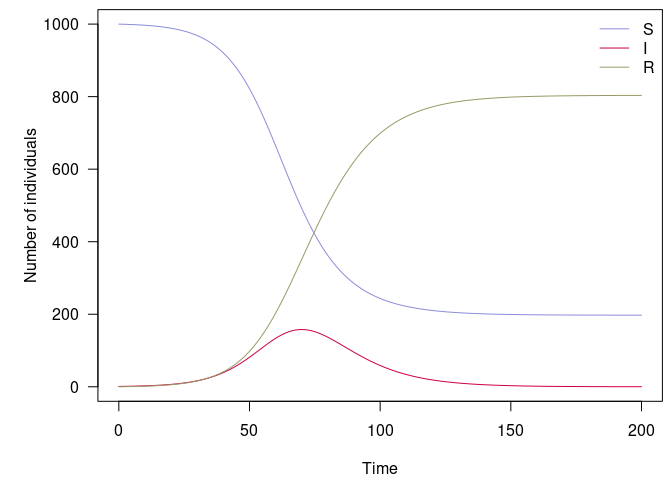An example of deterministic, discrete-time SIR model

## Stochastic SIR model

The stochastic equivalent of the previous model can be formulated in odin as follows:

path_sir_model_s <- system.file("examples/discrete_stochastic_sir.R", package = "odin")
## Core equations for transitions between compartments:
update(S) <- S - n_SI
update(I) <- I + n_SI - n_IR
update(R) <- R + n_IR

## Individual probabilities of transition:
p_SI <- 1 - exp(-beta * I / N) # S to I
p_IR <- 1 - exp(-gamma) # I to R

## Draws from binomial distributions for numbers changing between
## compartments:
n_SI <- rbinom(S, p_SI)
n_IR <- rbinom(I, p_IR)

## Total population size
N <- S + I + R

## Initial states:
initial(S) <- S_ini
initial(I) <- I_ini
initial(R) <- 0

## User defined parameters - default in parentheses:
S_ini <- user(1000)
I_ini <- user(1)
beta <- user(0.2)
gamma <- user(0.1)

We can use the same workflow as before to run the model, using 10 initial infected individuals (I_ini = 10):

sir_s_generator <- odin::odin(path_sir_model_s)
sir_s_generator
## <odin_model> object generator
##   Public:
##     initialize: function (..., user = list(...), use_dde = FALSE, unused_user_action = NULL)
##     ir: function ()
##     set_user: function (..., user = list(...), unused_user_action = NULL)
##     initial: function (step)
##     rhs: function (step, y)
##     update: function (step, y)
##     contents: function ()
##     transform_variables: function (y)
##     run: function (step, y = NULL, ..., use_names = TRUE)
##   Private:
##     ptr: NULL
##     use_dde: NULL
##     odin: NULL
##     variable_order: NULL
##     output_order: NULL
##     n_out: NULL
##     ynames: NULL
##     interpolate_t: NULL
##     cfuns: list
##     dll: discrete.stochastic.sir8ccbfa6f
##     user: I_ini S_ini beta gamma
##     registration: function ()
##     set_initial: function (step, y, use_dde)
##   Parent env: <environment: namespace:discrete.stochastic.sir8ccbfa6f>
##   Locked objects: TRUE
##   Locked class: FALSE
##   Portable: TRUE
x <- sir_s_generator$new(I_ini = 10) set.seed(1) x_res <- x$run(0:100)
par(mar = c(4.1, 5.1, 0.5, 0.5), las = 1)
matplot(x_res[, 1], x_res[, -1], xlab = "Time", ylab = "Number of individuals",
type = "l", col = sir_col, lty = 1)
legend("topright", lwd = 1, col = sir_col, legend = c("S", "I", "R"), bty = "n")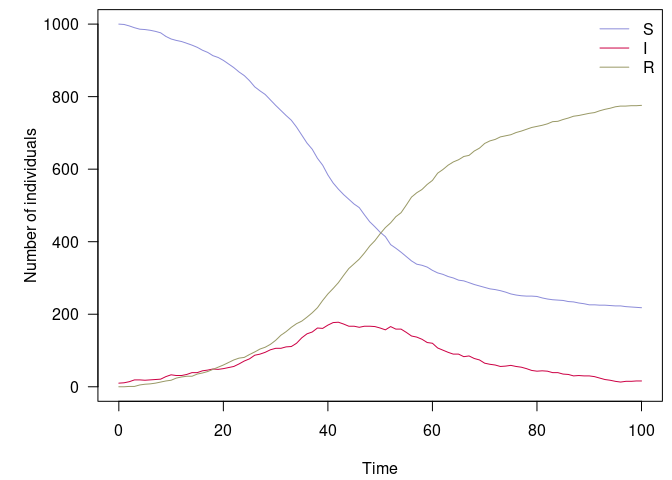An example of stochastic, discrete-time SIR model

This gives us a single stochastic realisation of the model, which is of limited interest. As an alternative, we can generate a large number of replicates using arrays for each compartment:

path_sir_model_s_a <- system.file("examples/discrete_stochastic_sir_arrays.R", package = "odin")
## Core equations for transitions between compartments:
update(S[]) <- S[i] - n_SI[i]
update(I[]) <- I[i] + n_SI[i] - n_IR[i]
update(R[]) <- R[i] + n_IR[i]

## Individual probabilities of transition:
p_SI[] <- 1 - exp(-beta * I[i] / N[i])
p_IR <- 1 - exp(-gamma)

## Draws from binomial distributions for numbers changing between
## compartments:
n_SI[] <- rbinom(S[i], p_SI[i])
n_IR[] <- rbinom(I[i], p_IR)

## Total population size
N[] <- S[i] + I[i] + R[i]

## Initial states:
initial(S[]) <- S_ini
initial(I[]) <- I_ini
initial(R[]) <- 0

## User defined parameters - default in parentheses:
S_ini <- user(1000)
I_ini <- user(1)
beta <- user(0.2)
gamma <- user(0.1)

## Number of replicates
nsim <- user(100)
dim(N) <- nsim
dim(S) <- nsim
dim(I) <- nsim
dim(R) <- nsim
dim(p_SI) <- nsim
dim(n_SI) <- nsim
dim(n_IR) <- nsim
sir_s_a_generator <- odin::odin(path_sir_model_s_a)
sir_s_a_generator
## <odin_model> object generator
##   Public:
##     initialize: function (..., user = list(...), use_dde = FALSE, unused_user_action = NULL)
##     ir: function ()
##     set_user: function (..., user = list(...), unused_user_action = NULL)
##     initial: function (step)
##     rhs: function (step, y)
##     update: function (step, y)
##     contents: function ()
##     transform_variables: function (y)
##     run: function (step, y = NULL, ..., use_names = TRUE)
##   Private:
##     ptr: NULL
##     use_dde: NULL
##     odin: NULL
##     variable_order: NULL
##     output_order: NULL
##     n_out: NULL
##     ynames: NULL
##     interpolate_t: NULL
##     cfuns: list
##     dll: discrete.stochastic.sir.arrays4ec523f1
##     user: I_ini S_ini beta gamma nsim
##     registration: function ()
##     set_initial: function (step, y, use_dde)
##   Parent env: <environment: namespace:discrete.stochastic.sir.arrays4ec523f1>
##   Locked objects: TRUE
##   Locked class: FALSE
##   Portable: TRUE
x <- sir_s_a_generator$new() set.seed(1) sir_col_transp <- paste0(sir_col, "66") x_res <- x$run(0:100)
par(mar = c(4.1, 5.1, 0.5, 0.5), las = 1)
matplot(x_res[, 1], x_res[, -1], xlab = "Time", ylab = "Number of individuals",
type = "l", col = rep(sir_col_transp, each = 100), lty = 1)
legend("left", lwd = 1, col = sir_col, legend = c("S", "I", "R"), bty = "n")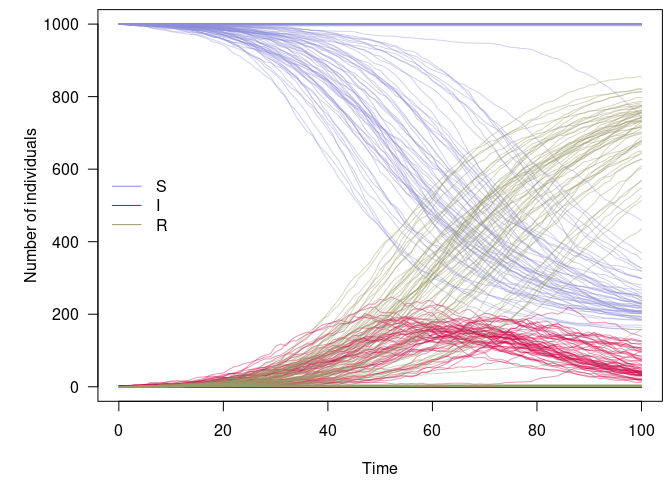100 replicates of a stochastic, discrete-time SIR model

## A stochastic SEIRDS model

This model is a more complex version of the previous one, which we will use to illustrate the use of all distributions mentioned in the first part: Binomial, Poisson and Multinomial.

The model is contains the following compartments:

• $$S$$: susceptibles
• $$E$$: exposed, i.e. infected but not yet contagious
• $$I_R$$: infectious who will survive
• $$I_D$$: infectious who will die
• $$R$$: recovered
• $$D$$: dead

There are no birth of natural death processes in this model. Parameters are:

• $$\beta$$: rate of infection
• $$\delta$$: rate at which symptoms appear (i.e inverse of mean incubation period)
• $$\gamma_R$$: recovery rate
• $$\gamma_D$$: death rate
• $$\mu$$: case fatality ratio (proportion of cases who die)
• $$\epsilon$$: import rate of infected individuals (applies to $$E$$ and $$I$$)
• $$\omega$$: rate waning immunity

The model will be written as:

$S_{t+1} = S_t - \beta \frac{S_t (I_{R,t} + I_{D,t})}{N_t} + \omega R_t$

$E_{t+1} = E_t + \beta \frac{S_t (I_{R,t} + I_{D,t})}{N_t} - \delta E_t + \epsilon$

$I_{R,t+1} = I_{R,t} + \delta (1 - \mu) E_t - \gamma_R I_{R,t} + \epsilon$

$I_{D,t+1} = I_{D,t} + \delta \mu E_t - \gamma_D I_{D,t} + \epsilon$

$R_{t+1} = R_t + \gamma_R I_{R,t} - \omega R_t$

$D_{t+1} = D_t + \gamma_D I_{D,t}$

The formulation of the model in odin is:

path_seirds_model <- system.file("examples/discrete_stochastic_seirds.R", package = "odin")
## Core equations for transitions between compartments:
update(S) <- S - n_SE + n_RS
update(E) <- E + n_SE - n_EI + n_import_E
update(Ir) <- Ir + n_EIr - n_IrR
update(Id) <- Id + n_EId - n_IdD
update(R) <- R + n_IrR - n_RS
update(D) <- D + n_IdD

## Individual probabilities of transition:
p_SE <- 1 - exp(-beta * I / N)
p_EI <-  1 - exp(-delta)
p_IrR <- 1 - exp(-gamma_R) # Ir to R
p_IdD <- 1 - exp(-gamma_D) # Id to d
p_RS <- 1 - exp(-omega) # R to S

## Draws from binomial distributions for numbers changing between
## compartments:
n_SE <- rbinom(S, p_SE)
n_EI <- rbinom(E, p_EI)

n_EIrId[] <- rmultinom(n_EI, p)
p <- 1 - mu
p <- mu
dim(p) <- 2
dim(n_EIrId) <- 2
n_EIr <- n_EIrId
n_EId <- n_EIrId
n_IrR <- rbinom(Ir, p_IrR)
n_IdD <- rbinom(Id, p_IdD)

n_RS <- rbinom(R, p_RS)

n_import_E <- rpois(epsilon)

## Total population size, and number of infecteds
I <- Ir + Id
N <- S + E + I + R + D

## Initial states
initial(S) <- S_ini
initial(E) <- E_ini
initial(Id) <- 0
initial(Ir) <- 0
initial(R) <- 0
initial(D) <- 0

## User defined parameters - default in parentheses:
S_ini <- user(1000) # susceptibles
E_ini <- user(1) # infected
beta <- user(0.3) # infection rate
delta <- user(0.3) # inverse incubation
gamma_R <- user(0.08) # recovery rate
gamma_D <- user(0.12) # death rate
mu <- user(0.7) # CFR
omega <- user(0.01) # rate of waning immunity
epsilon <- user(0.1) # import case rate
seirds_generator <- odin::odin(path_seirds_model)
seirds_generator
## <odin_model> object generator
##   Public:
##     initialize: function (..., user = list(...), use_dde = FALSE, unused_user_action = NULL)
##     ir: function ()
##     set_user: function (..., user = list(...), unused_user_action = NULL)
##     initial: function (step)
##     rhs: function (step, y)
##     update: function (step, y)
##     contents: function ()
##     transform_variables: function (y)
##     run: function (step, y = NULL, ..., use_names = TRUE)
##   Private:
##     ptr: NULL
##     use_dde: NULL
##     odin: NULL
##     variable_order: NULL
##     output_order: NULL
##     n_out: NULL
##     ynames: NULL
##     interpolate_t: NULL
##     cfuns: list
##     dll: discrete.stochastic.seirdsf8855d06
##     user: E_ini S_ini beta delta epsilon gamma_D gamma_R mu omega
##     registration: function ()
##     set_initial: function (step, y, use_dde)
##   Parent env: <environment: namespace:discrete.stochastic.seirdsf8855d06>
##   Locked objects: TRUE
##   Locked class: FALSE
##   Portable: TRUE
x <- seirds_generator$new() seirds_col <- c("#8c8cd9", "#e67300", "#d279a6", "#ff4d4d", "#999966", "#660000") set.seed(1) x_res <- x$run(0:365)
par(mar = c(4.1, 5.1, 0.5, 0.5), las = 1)
matplot(x_res[, 1], x_res[, -1], xlab = "Time", ylab = "Number of individuals",
type = "l", col = seirds_col, lty = 1)
legend("left", lwd = 1, col = seirds_col, legend = c("S", "E", "Ir", "Id", "R", "D"), bty = "n")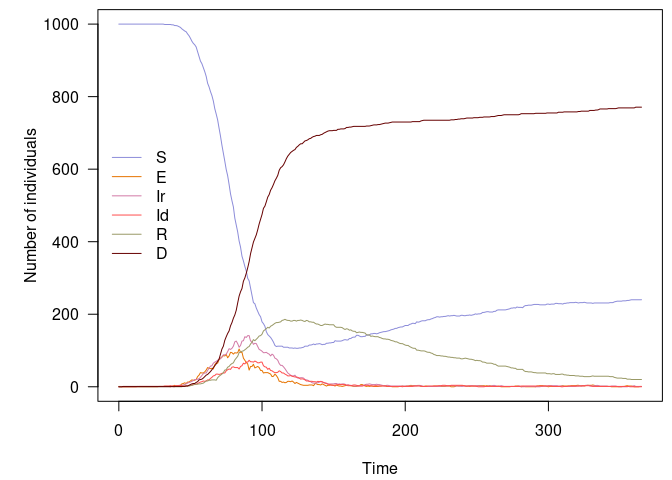Several runs can be obtained without rewriting the model, for instance, to get 100 replicates:

x_res <- as.data.frame(replicate(100, x$run(0:365)[, -1])) dim(x_res) ##  366 600 x_res[1:6, 1:10] ## S.1 E.1 Id.1 Ir.1 R.1 D.1 S.2 E.2 Id.2 Ir.2 ## 1 1000 1 0 0 0 0 1000 1 0 0 ## 2 1000 1 0 0 0 0 1000 1 0 0 ## 3 1000 0 0 1 0 0 1000 2 0 0 ## 4 1000 0 0 1 0 0 1000 1 1 0 ## 5 1000 0 0 1 0 0 1000 1 1 0 ## 6 1000 0 0 1 0 0 1000 0 2 0 seirds_col_transp <- paste0(seirds_col, "1A") par(mar = c(4.1, 5.1, 0.5, 0.5), las = 1) matplot(0:365, x_res, xlab = "Time", ylab = "Number of individuals", type = "l", col = rep(seirds_col_transp, 100), lty = 1) legend("right", lwd = 1, col = seirds_col, legend = c("S", "E", "Ir", "Id", "R", "D"), bty = "n")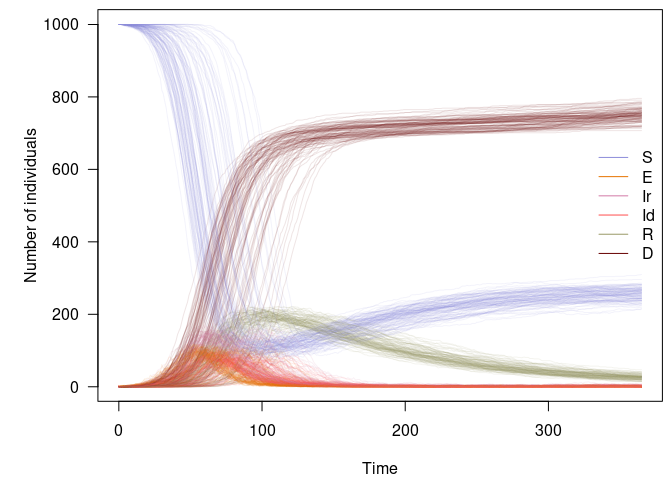100 replicates of a stochastic, discrete-time SEIRDS model It is then possible to explore the behaviour of the model using a simple function: check_model <- function(n = 50, t = 0:365, alpha = 0.2, ..., legend_pos = "topright") { model <- seirds_generator$new(...)
col <- paste0(seirds_col, "33")

res <- as.data.frame(replicate(n, model\$run(t)[, -1]))
on.exit(par(opar))
par(mar = c(4.1, 5.1, 0.5, 0.5), las = 1)
matplot(t, res, xlab = "Time", ylab = "",  type = "l",
col = rep(col, n), lty = 1)
mtext("Number of individuals", side = 2, line = 3.5, las = 3, cex = 1.2)
legend(legend_pos, lwd = 1, col = seirds_col,
legend = c("S", "E", "Ir", "Id", "R", "D"), bty = "n")
}

This is a sanity check with a null infection rate and no imported case:

check_model(beta = 0, epsilon = 0)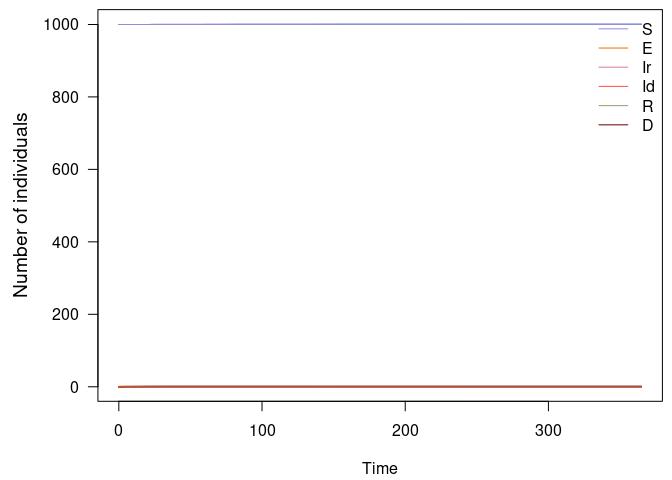Stochastic SEIRDS model: sanity check with no infections

Another easy case: no importation, no waning immunity:

check_model(epsilon = 0, omega = 0)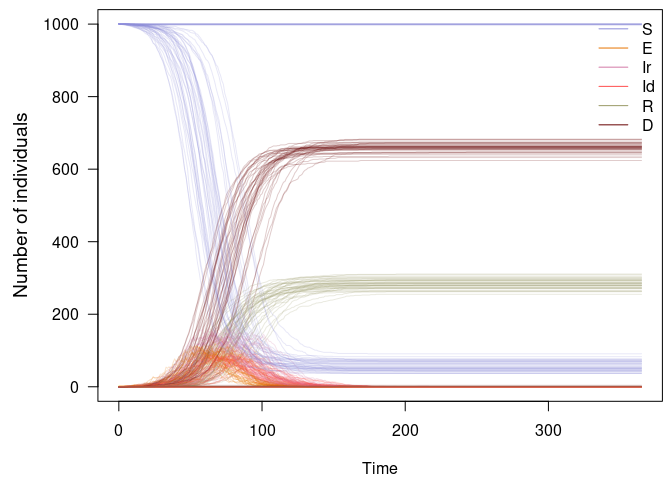Stochastic SEIRDS model: no importation or waning immunity

A more nuanced case: persistence of the disease with limited import, waning immunity, low severity, larger population:

check_model(t = 0:(365 * 3), epsilon = 0.1, beta = .2, omega = .01,
mu = 0.005, S_ini = 1e5)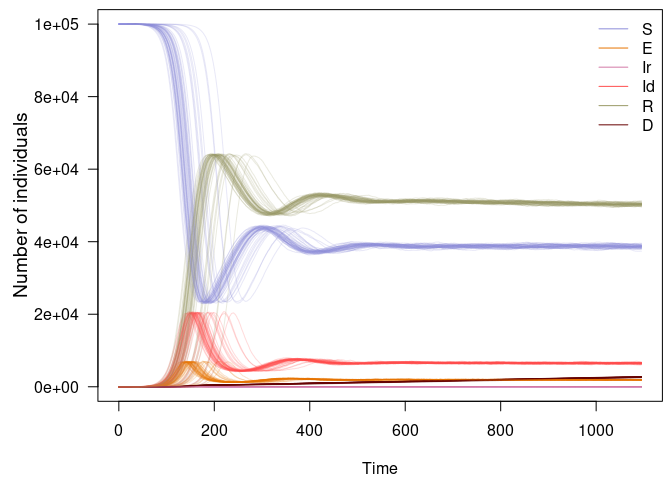Stochastic SEIRDS model: endemic state in a larger population